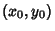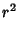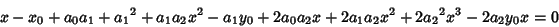Find the minimum distance between a point in the planeand a quadratic Plane Curve(1)

The square of the distance is(2)

Minimizing the distance squared is the equivalent to minimizing the distance (sinceandhave minima at the same point), so take(3)(4)(5)

Minimizing the distance therefore requires solution of a Cubic Equation.